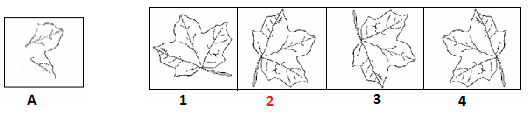# Embedded Images - Non Verbal Reasoning questions

## Embedded Images - Non Verbal Reasoning questions

Q1. Find out the alternative figure which contains figure (A) as its part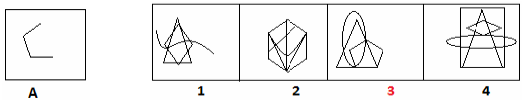Q2. Find out the alternative figure which contains figure (A) as its part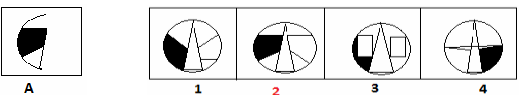Q3. Find out the alternative figure which contains figure (A) as its part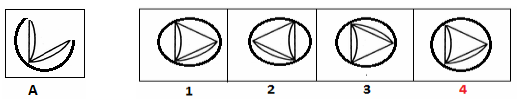Q4. Find out the alternative figure which contains figure (A) as its part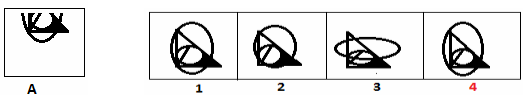Q5. Find out the alternative figure which contains figure (A) as its part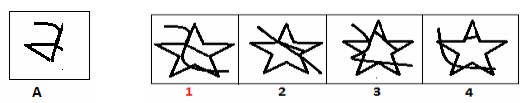Q6. Find out the alternative figure which contains figure (A) as its part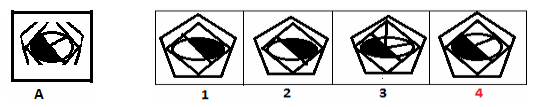Q7. Find out the alternative figure which contains figure (A) as its part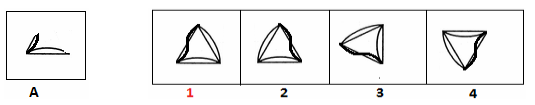Q8. Find out the alternative figure which contains figure (A) as its part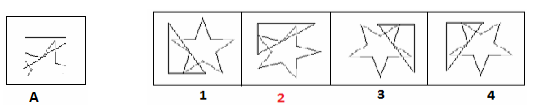Q9. Find out the alternative figure which contains figure (A) as its part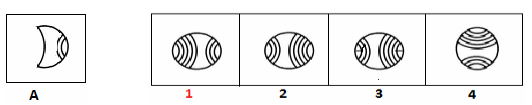Q10. Find out the alternative figure which contains figure (A) as its part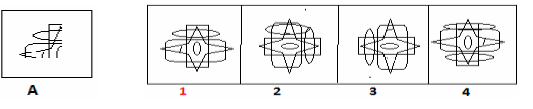Q11. Find out the alternative figure which contains figure (A) as its part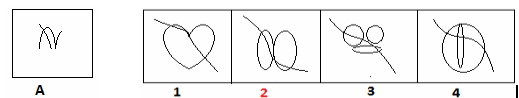Q12. Find out the alternative figure which contains figure (A) as its part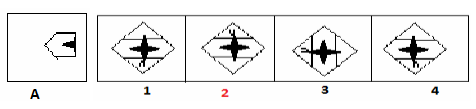Q13. Find out the alternative figure which contains figure (A) as its part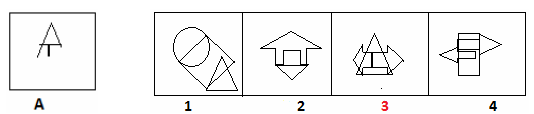Q14. Find out the alternative figure which contains figure (A) as its part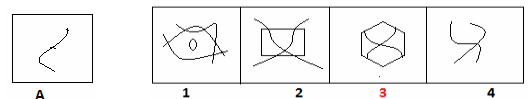Q15. Find out the alternative figure which contains figure (A) as its part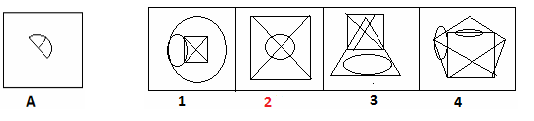Q16. Find out the alternative figure which contains figure (A) as its part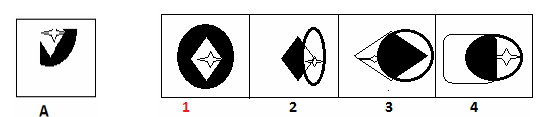Q17. Find out the alternative figure which contains figure (A) as its part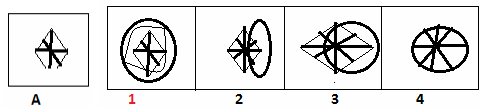Q18. Find out the alternative figure which contains figure (A) as its part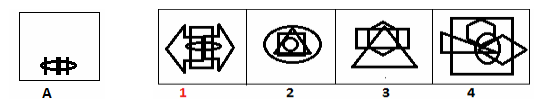Q19. Find out the alternative figure which contains figure (A) as its part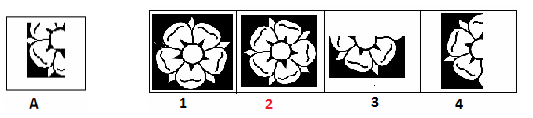Q20. Find out the alternative figure which contains figure (A) as its part